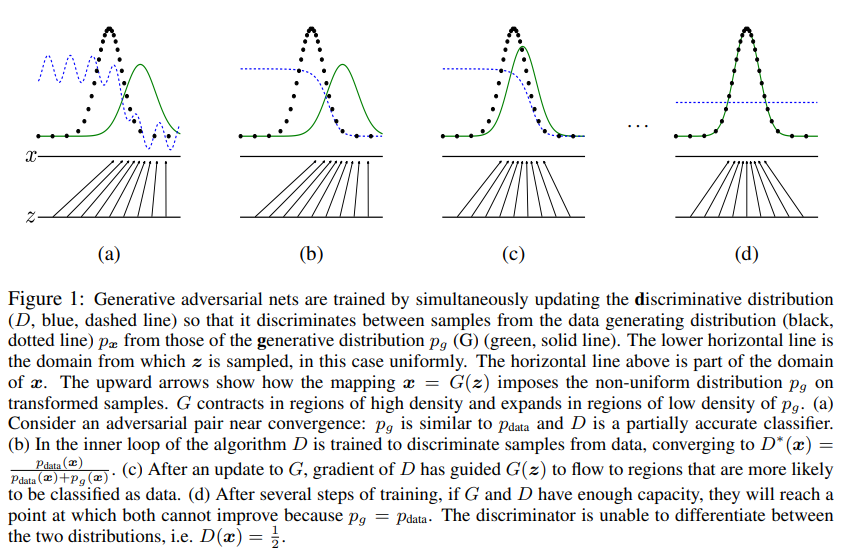# The Basic : Generative Adversarial Networks (GANs)

taejinjeong·2021년 6월 28일
0

목록 보기
1/1

## The Concepts of GANs

### Discriminative Model vs Generative Model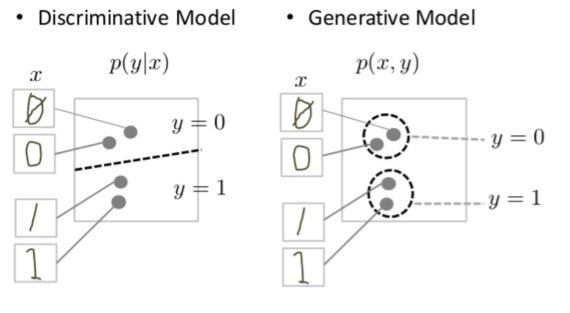The comparison of Discriminative Model and Generative Model.
The Discriminative Model tries to draw a line in the data space to distinguish the data.
In contrast, the Generative Model tries to produce data which fall close to their real counterparts in the data space.

### The Basic Idea of Generative Adversarial Networks (GANs)

The Generative Adversarial Networks (GANs) consists of two parts; Generator and Discriminator.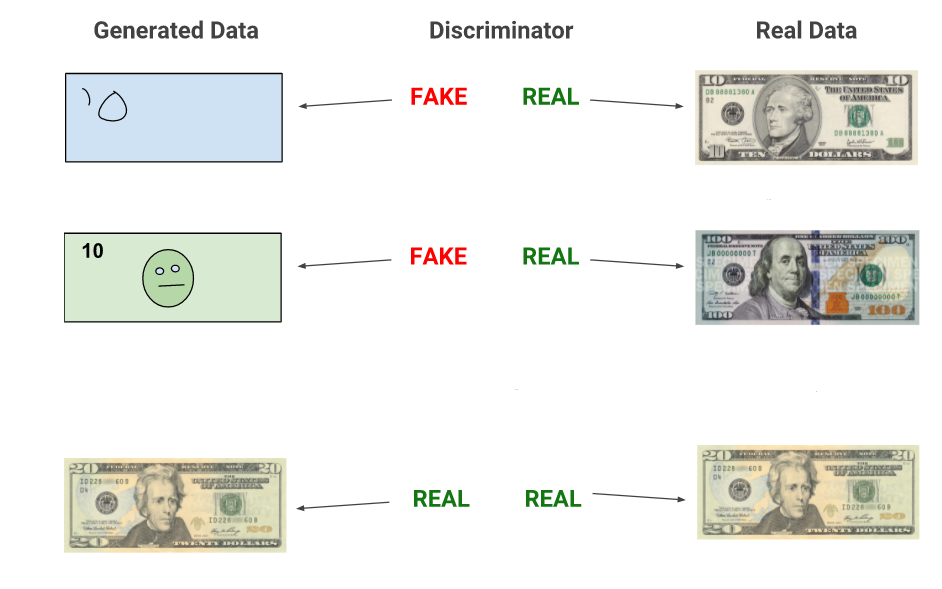The generator tries to create samples that are intended to come from the same distribution as the training data, while the discriminator tries to examine samples to determine whethere they are real (coming from the sample dataset) or fake (coming from the generator) as shown above.
Since those two networks pursue their goals without controlling other actions interactively(decieving the discriminator / detecting the output created by the generator), this framework can be interpreted as Game Theory: two-player minimax Game and the solution will land to a Nash Equilibria.

### Game Theory: Minimax Game

The Minimax Game is a decision rule for minimizing the possible loss for a worst case scenario.
The solution of the game is to maximize the minimum gain, which is referred to as maximin value.
The maximin value is the highest value that the player can be sure to get without knowing the actions of the other players defined as below.
$v_i = \max_{a_i}\min_{a_{-i}}v_i(a_i,a_{-i})$
where :

• $i$ is the index of the player of interest
• $-i$ denotes all other players except player $i$
• $a_i$ is the action taken by player $i$
• $a_{-i}$ denotes the actions taken by all other players
• $v_i$ is the value function of player $i$

The solution to a game is a Nash Equilibria which is a tuple ($\theta^D, \theta^G$) that is a local minimum of $J^D$ with respect to $\theta^D$ and a local minimum of $J^G$ with respect to $\theta^G$.

## The Theory of GANs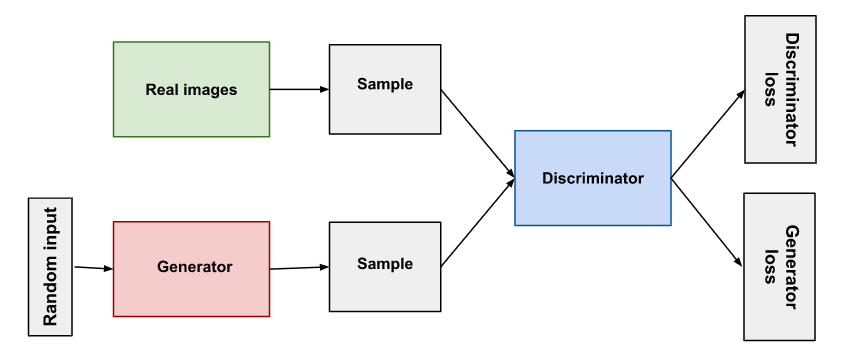The overview of GANs architecture consists of two networks, generator $G$ and discriminator $D$, each of which is differentiable both with respect to its inputs, $z$ for $G$ and $x$ for $D$, and with respect to its parameters, $\theta^D$ for $G$ and $\theta^D$ for $D$

### Generator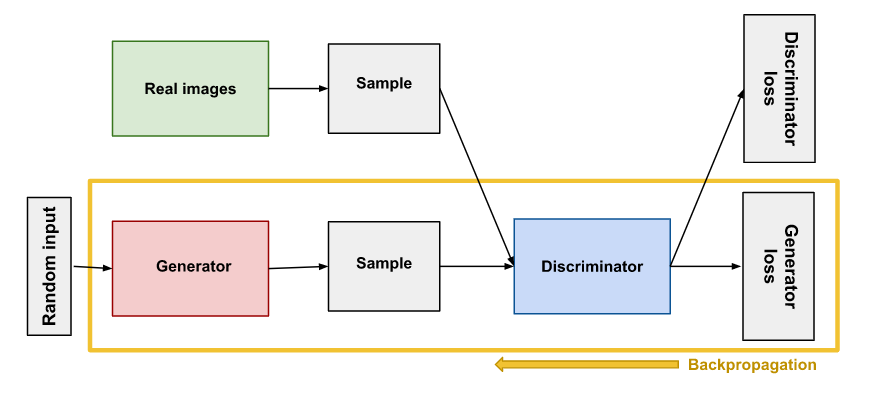The generator is simply a differentiable function $G$ with input $z$, the prior probability.
When $z$ is sampled from some simple prior distribution, $G(z)$ yeilds a sample of $x$ drawm from $p_{model}$.
The inputs to the function $G$ do not need to correspond to inputs to the first layer of the deep neural net; inputs may be provided at any point throughout the network.
If we want $p_{model}$ to have full support on $x$ space we need the dimension of $z$ to be at least as large as the dimension of $x$, and $G$ must be differentiable, but those are the only requirements.

### Discriminator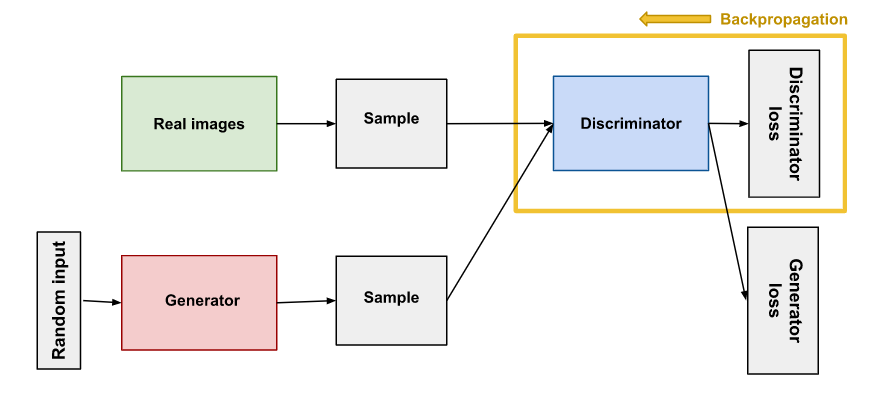The discriminator is simply a differentiable classifier function $D$.
The purpose of the discriminator is to distinguish the real data from the data created by the generator ( $D(x)=1$ and $D(G(z))=0$ ).
The inputs to the function $D$ come from two different sources; one is from examples of $x$ randomly sampled from the training set and the other is from the data created by generator.

### Cost Functions

To build a Cost Functions for GANs, it is essential to know maximum likelihood estimation and minimax problem.

#### Maximum likelihood estimation

The basic idea of maximum likelihood is to define a model that provides an estimate of a probability distribution, parameterized by parameters $\theta$.
The likelihood as the probability that the model assigns to the training data is refered as below.

$\prod_{i = 1}^{m} p_{model}(x^i,\theta)$, for a dataset containing $m$ training examples $x^i$

The principle of maximum likelihood simply says to choose the parameters for the model that maximize the likelihood of the training data.

$\theta^* = \arg\max_\theta\prod_{i=1}^m p_{model}(x^i,\theta)$
$\ \ \ \ \ = \arg\max_\theta\log\prod_{i=1}^m p_{model}(x^i,\theta)$
$\ \ \ \ \ = \arg\max_\theta\sum_{i=1}^m\log p_{model}(x^i,\theta)$

The maximum likelihood estimation would be interpreted as minimizing the Kullback-Leibler Divergence(KL divergence) between the data generating distribution and the model as below.

$\theta^*=\arg\min_\theta D_{KL}(p_{data}(x)||p_{model}(x;\theta))$

#### Kullback-Leibler Divergence (KL Divergence)

In mathematical statistics, the Kullback–Leibler divergence, $D_{KL}$ (also called relative entropy), is a measure of how one probability distribution is different from a second, reference probability distribution.
Consider two probability distributions $P$ and $Q$ where, usually, $P$ represents the data, the observations, or a measured probability distribution and distribution $Q$ represents instead a theory, a model, a description or an approximation of $P$.
The Kullback–Leibler divergence is then interpreted as the average difference of the number of bits required for encoding samples of $P$ using a code optimized for $Q$ rather than one optimized for $P$.
For discrete probability distributions $P$ and $Q$ defined on the same probability space, $X$, the relative entropy or KL divergence from $Q$ to $P$ is defined as below.

$D_{KL}(P||Q)=\sum_{x\in X}P(x)\log (\frac{P(x)}{Q(x)})$ where, $x$ is discrete
$D_{KL}(P||Q)=\int_x p(x)\log(\frac{p(x)}{q(x)})dx$ where, $x$ is continuous.

In other words, it is the expectation of the logarithmic difference between the probabilities $P$ and $Q$, where the expectation is taken using the probabilities $P$.

#### Jensen-Shannon Divergence

In probability theory and statistics, the Jensen–Shannon divergence is a method of measuring the similarity between two probability distributions.
It is based on the Kullback–Leibler divergence, with some notable (and useful) differences, including that it is symmetric and it always has a finite value.

$JSD(P||Q)=\frac{1}{2}D(P||M)+\frac{1}{2}D(Q||M)$ where $M=\frac{1}{2}(P+M)$

#### Objective Function (Minimax Loss)

Since GANs consists of two player, generator and discriminator which try to pursue their goals without knowing each other's actions, The objective function can be set up based on minimax game as below.
$\min_G\max_DV(D,G) = \mathbb{E}_{x\sim p_{data}}[\log D(x)] + \mathbb{E}_{z\sim p_{z}}[\log(1-D(G(z)))]$

#### Objective Function in the Perspective of Discriminator

First, consider the optimal discriminator, $D$ for any given generator, $G$.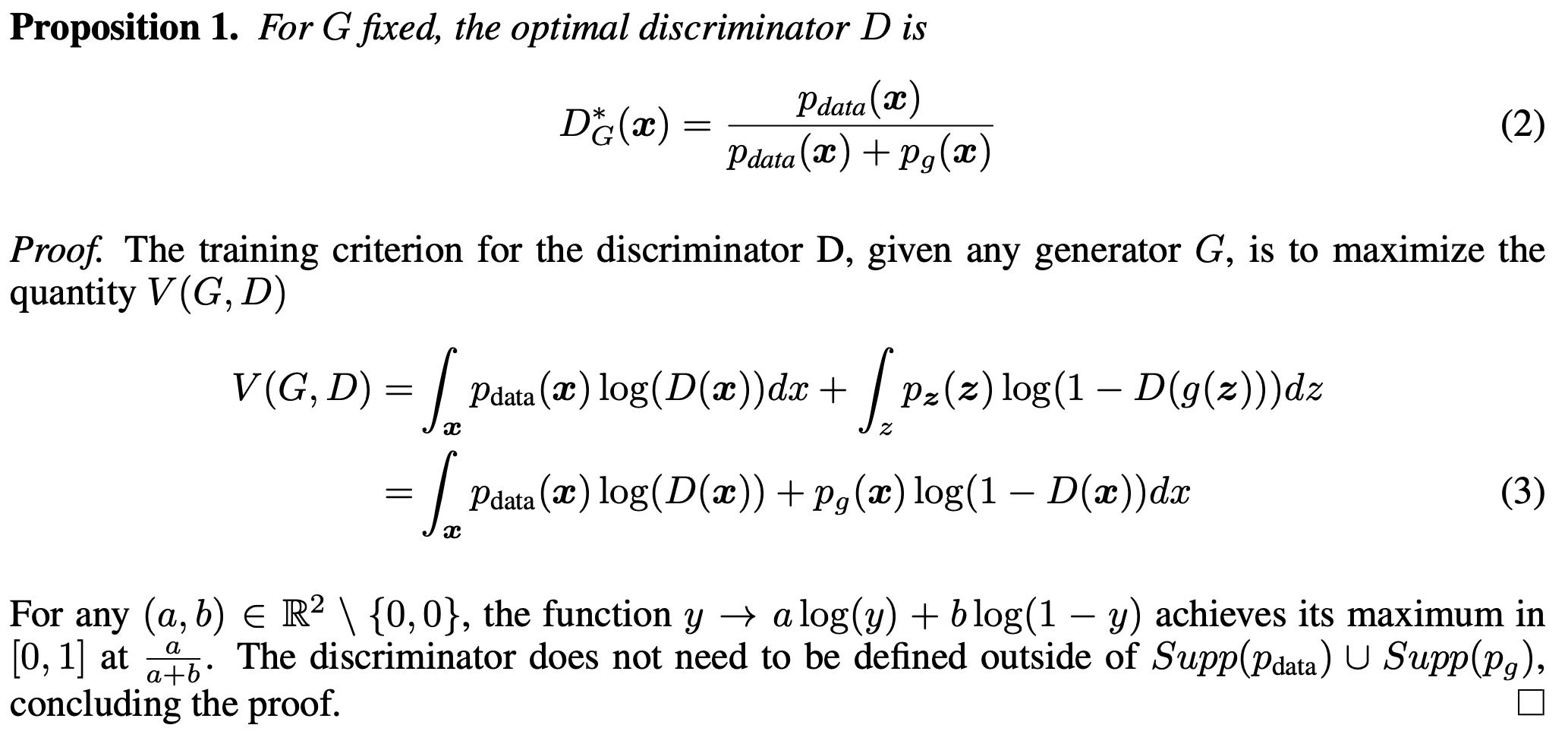#### Objective Function in the Perspective of Generator

Since the training objective for $D$ can be interpreted as maximizing the log-likelihood for estimating the conditional probability $P(Y=y|x)$, where Y indicates whether $x$ comes from $p_{data}$ (with $y=1$) or from $p_g$ (with $y=0$), the cost function, $C(G)$ reformulated as below. And, with the cost function, $C(G)$, the global minimum is achieved when $p_g=p_{data}$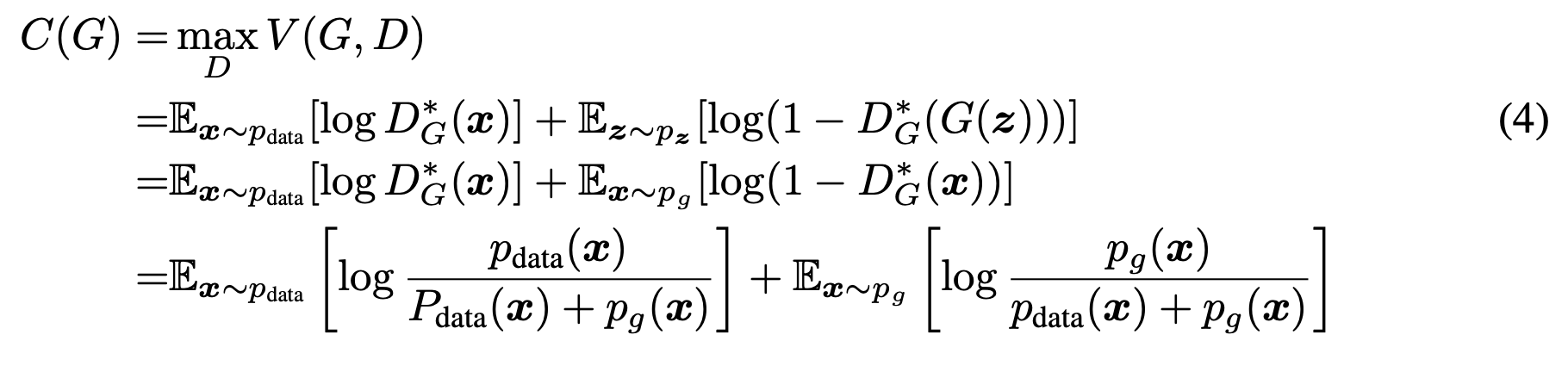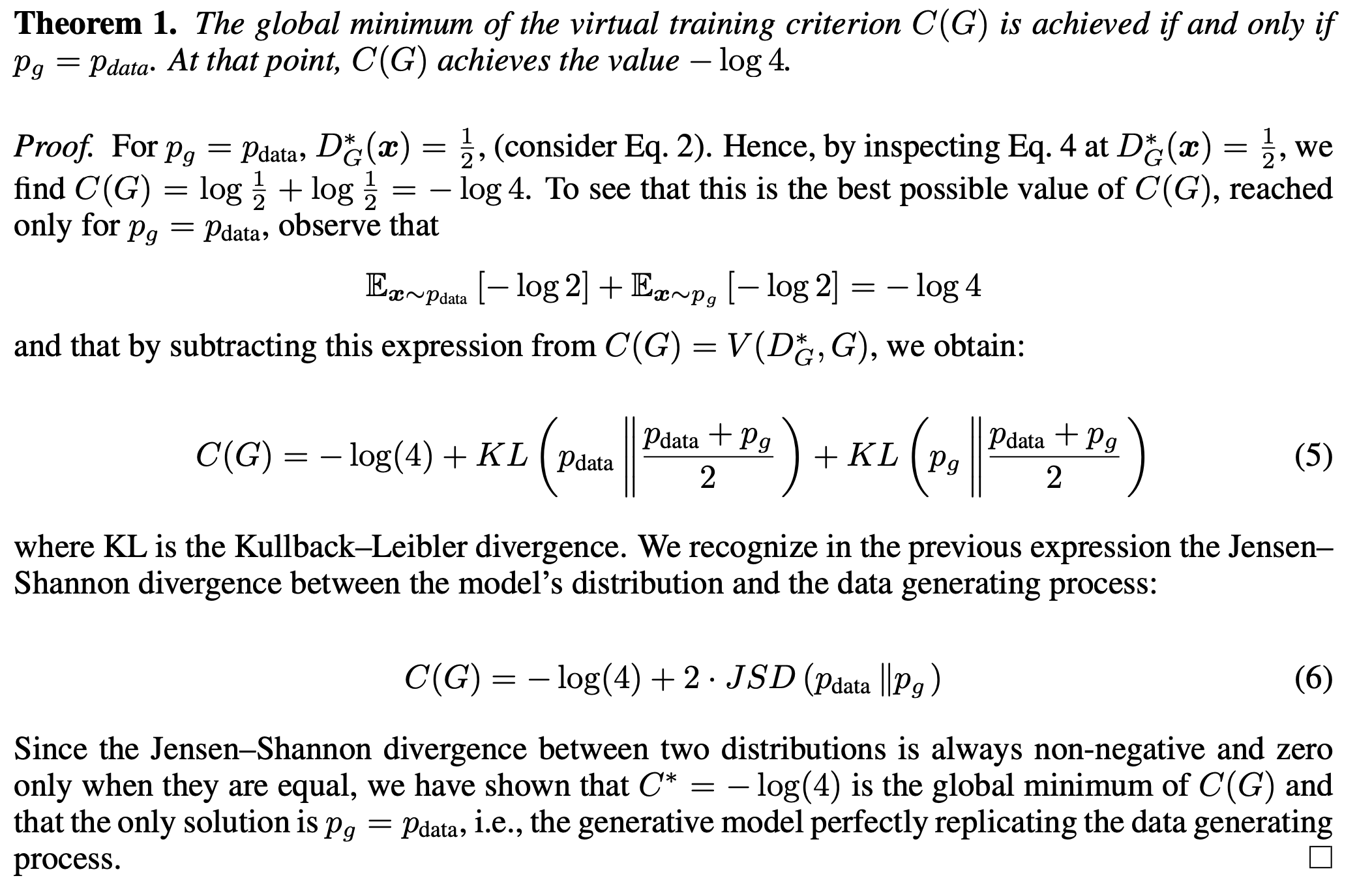Detailed infromation for the proof of Theorem 1
The value function $V(G,D)$ with the optimal disciminator $D^{*}_{G}(x)$ can be interpreted as cost function $C(G)$ for discriminator with fixed $G$.
$C(G) = \max_DV(G,D) = V(G,D^{*}_G)$

$\ \ \ \ \ \ \ \ \ \ \ = \int_x p_{data}(x) \log(D_{G}^{*}(x)) + p_g(x) \log(1-D_{G}^{*}(x))dx$

$\ \ \ \ \ \ \ \ \ \ \ = \int_x p_{data}(x) \log(\frac{p_{data}(x)}{p_{data}(x) + p_{g}(x)}) + p_g(x) \log(\frac{p_{g}(x)}{p_{g}(x) + p_{data}(x)})dx$

$\ \ \ \ \ \ \ \ \ \ \ = -\log(4) + \int_x p_{data}(x) \log(\frac{p_{data}(x)}{p_{data}(x) + p_{g}(x)})dx + \log(2)$
$\ \ \ \ \ \ \ \ \ \ \ \ \ \ \ \ \ \ \ \ +\int_x p_g(x) \log(\frac{p_{g}(x)}{p_{g}(x) + p_{data}(x)})dx + \log(2)$

$\ \ \ \ \ \ \ \ \ \ \ = -\log(4) + \int_x p_{data}(x) \log(\frac{2p_{data}(x)}{p_{data}(x) + p_{g}(x)}) + p_g(x) \log(\frac{2p_{g}(x)}{p_{g}(x) + p_{data}(x)})dx$

$\ \ \ \ \ \ \ \ \ \ \ = -\log(4) + \int_x p_{data}(x) (\log(p_{data}(x)) + \log(\frac{p_{data}(x)+p_{g}(x)}{2}))$
$\ \ \ \ \ \ \ \ \ \ \ \ \ \ \ \ \ \ \ \ +p_g(x) (\log(p_{g}(x)) + \log(\frac{p_{data}(x)+p_{g}(x)}{2}))dx$

$\ \ \ \ \ \ \ \ \ \ \ = -\log(4) + KL(p_{data}||\frac{p_{data}+p_{g}}{2}) + KL(p_{g}||\frac{p_{data}+p_{g}}{2})$

$\ \ \ \ \ \ \ \ \ \ \ = -\log(4) + 2 \ \cdot\ JSD(p_{data}||p_g)$

Thus, $C(G)$ is achieved the global minimum at $p_g=p_{data}$ with the value $-\log(4)$

### Training Process and Convergence of Algorithm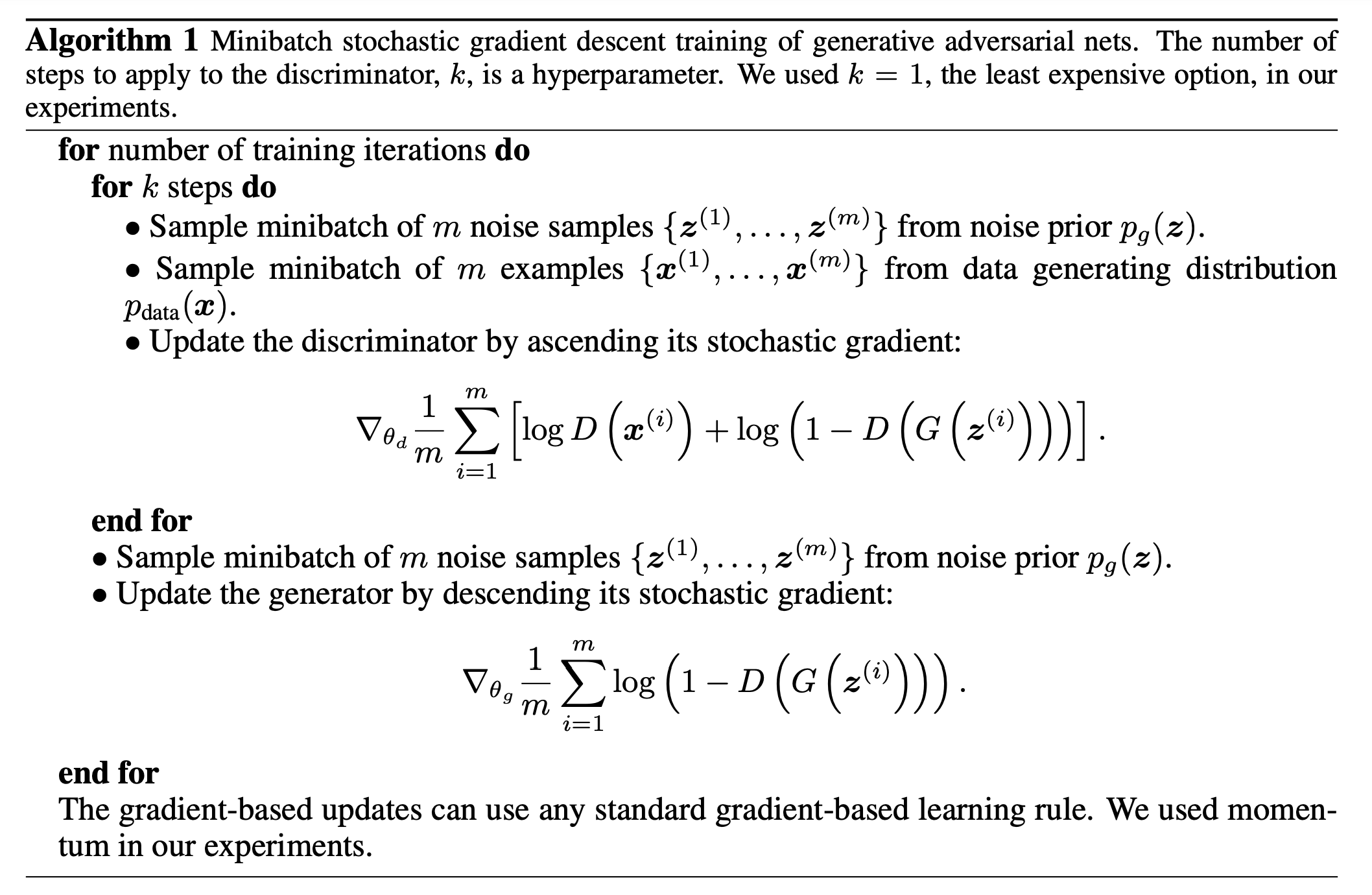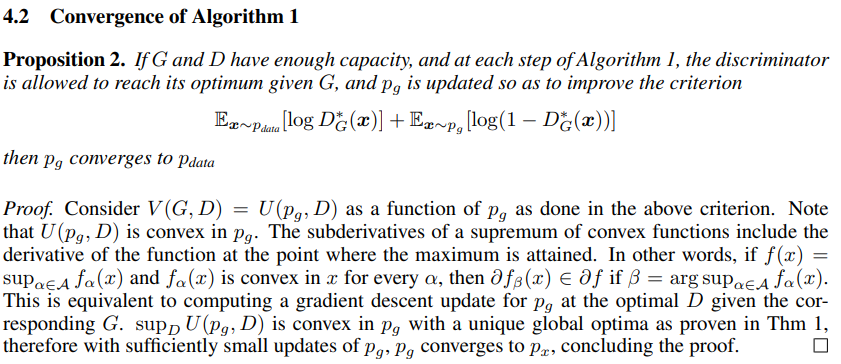The overview of the Algorithm1 is given below.